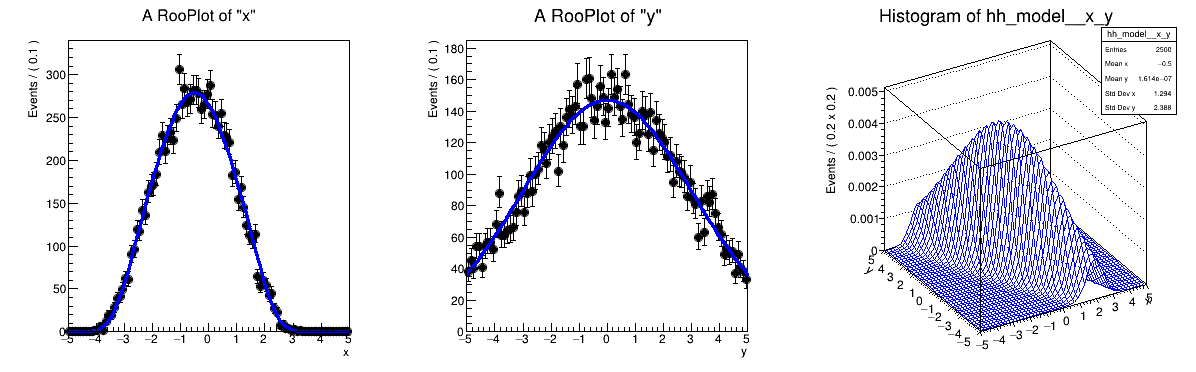# Rf 3 0 5_Condcorrprod¶

Multidimensional models: multi-dimensional pdfs with conditional pdfs in product

pdf = gauss(x,f(y),sx | y ) * gauss(y,ms,sx) with f(y) = a0 + a1*y

Author: Clemens Lange, Wouter Verkerke (C++ version)
This notebook tutorial was automatically generated with ROOTBOOK-izer from the macro found in the ROOT repository on Saturday, November 28, 2020 at 10:45 AM.

In :
import ROOT

Welcome to JupyROOT 6.23/01


## Create conditional pdf gx(x|y)¶

Create observables

In :
x = ROOT.RooRealVar("x", "x", -5, 5)
y = ROOT.RooRealVar("y", "y", -5, 5)

RooFit v3.60 -- Developed by Wouter Verkerke and David Kirkby
Copyright (C) 2000-2013 NIKHEF, University of California & Stanford University



Create function f(y) = a0 + a1*y

In :
a0 = ROOT.RooRealVar("a0", "a0", -0.5, -5, 5)
a1 = ROOT.RooRealVar("a1", "a1", -0.5, -1, 1)
fy = ROOT.RooPolyVar("fy", "fy", y, ROOT.RooArgList(a0, a1))


Create gaussx(x,f(y),sx)

In :
sigmax = ROOT.RooRealVar("sigma", "width of gaussian", 0.5)
gaussx = ROOT.RooGaussian(
"gaussx", "Gaussian in x with shifting mean in y", x, fy, sigmax)

[#0] WARNING:InputArguments -- The parameter 'sigma' with range [-1e+30, 1e+30] of the RooGaussian 'gaussx' exceeds the safe range of (0, inf). Advise to limit its range.


## Create pdf gy(y)¶

Create gaussy(y,0,5)

In :
gaussy = ROOT.RooGaussian(
"gaussy",
"Gaussian in y",
y,
ROOT.RooFit.RooConst(0),
ROOT.RooFit.RooConst(3))


## Create product gx(x|y)*gy(y)¶

Create gaussx(x,sx|y) * gaussy(y)

In :
model = ROOT.RooProdPdf(
"model",
"gaussx(x|y)*gaussy(y)",
ROOT.RooArgSet(gaussy),
ROOT.RooFit.Conditional(
ROOT.RooArgSet(gaussx),
ROOT.RooArgSet(x)))


## Sample, fit and plot product pdf¶

Generate 1000 events in x and y from model

In :
data = model.generate(ROOT.RooArgSet(x, y), 10000)


Plot x distribution of data and projection of model x = Int(dy) model(x,y)

In :
xframe = x.frame()
data.plotOn(xframe)
model.plotOn(xframe)

Out:
<cppyy.gbl.RooPlot object at 0x7efdc40>
[#1] INFO:Plotting -- RooAbsReal::plotOn(model) plot on x integrates over variables (y)
[#1] INFO:NumericIntegration -- RooRealIntegral::init([gaussy_NORM[y]_X_gaussx_NORM[x]]_Int[y]) using numeric integrator RooIntegrator1D to calculate Int(y)


Plot x distribution of data and projection of model y = Int(dx) model(x,y)

In :
yframe = y.frame()
data.plotOn(yframe)
model.plotOn(yframe)

Out:
<cppyy.gbl.RooPlot object at 0x809b3e0>
[#1] INFO:Plotting -- RooAbsReal::plotOn(model) plot on y integrates over variables (x)


Make two-dimensional plot in x vs y

In :
hh_model = model.createHistogram("hh_model", x, ROOT.RooFit.Binning(
50), ROOT.RooFit.YVar(y, ROOT.RooFit.Binning(50)))
hh_model.SetLineColor(ROOT.kBlue)


Make canvas and draw ROOT.RooPlots

In :
c = ROOT.TCanvas("rf305_condcorrprod", "rf05_condcorrprod", 1200, 400)
c.Divide(3)
c.cd(1)
xframe.GetYaxis().SetTitleOffset(1.6)
xframe.Draw()
c.cd(2)
yframe.GetYaxis().SetTitleOffset(1.6)
yframe.Draw()
c.cd(3)
hh_model.GetZaxis().SetTitleOffset(2.5)
hh_model.Draw("surf")

c.SaveAs("rf305_condcorrprod.png")

Info in <TCanvas::Print>: png file rf305_condcorrprod.png has been created


Draw all canvases

In :
from ROOT import gROOT
gROOT.GetListOfCanvases().Draw()